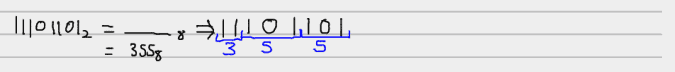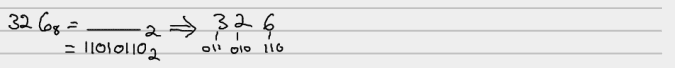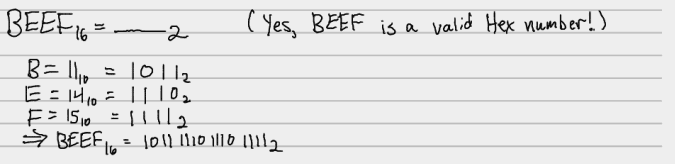# (guest@joequery.me)~ $| # Converting Oct/Hex to Binary ## Converting Binary to Octal Group the digits by three, starting from the right, and convert the sections to octal.## Converting Octal to Binary Simply expand each number into its binary equivalent and squish the results together.## Converting Binary to Hex First, recall counting to 15 in hex (the base 16 number system) is as follows: 1, 2, 3, 4, 5, 6, 7, 8, 9, A, B, C, D, E, F So$47_{10} = (16^1 \times 2) + (16^0 \times 15) = 2F_{16}\$

Now, to convert binary to Hex, group the digits by four, starting from the right, and convert the decimal equivalent of the grouping to hex.## Converting Hex to Binary

Similarly to octal to binary, simply take each number into its binary equivalent and squish the results together.## Tagged as computer science

Date published - November 24, 2013